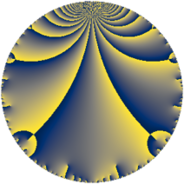# Properties

 Label 105.4.aLevel $105$ Weight $4$ Character orbit 105.a Rep. character $\chi_{105}(1,\cdot)$ Character field $\Q$ Dimension $12$ Newform subspaces $7$ Sturm bound $64$ Trace bound $2$

# Related objects

## Defining parameters

 Level: $$N$$ $$=$$ $$105 = 3 \cdot 5 \cdot 7$$ Weight: $$k$$ $$=$$ $$4$$ Character orbit: $$[\chi]$$ $$=$$ 105.a (trivial) Character field: $$\Q$$ Newform subspaces: $$7$$ Sturm bound: $$64$$ Trace bound: $$2$$ Distinguishing $$T_p$$: $$2$$

## Dimensions

The following table gives the dimensions of various subspaces of $$M_{4}(\Gamma_0(105))$$.

Total New Old
Modular forms 52 12 40
Cusp forms 44 12 32
Eisenstein series 8 0 8

The following table gives the dimensions of the cuspidal new subspaces with specified eigenvalues for the Atkin-Lehner operators and the Fricke involution.

$$3$$$$5$$$$7$$FrickeDim.
$$+$$$$+$$$$+$$$$+$$$$2$$
$$+$$$$-$$$$+$$$$-$$$$2$$
$$+$$$$-$$$$-$$$$+$$$$2$$
$$-$$$$+$$$$+$$$$-$$$$2$$
$$-$$$$+$$$$-$$$$+$$$$2$$
$$-$$$$-$$$$+$$$$+$$$$2$$
Plus space$$+$$$$8$$
Minus space$$-$$$$4$$

## Trace form

 $$12 q - 4 q^{2} + 56 q^{4} + 12 q^{6} - 28 q^{7} + 36 q^{8} + 108 q^{9} + O(q^{10})$$ $$12 q - 4 q^{2} + 56 q^{4} + 12 q^{6} - 28 q^{7} + 36 q^{8} + 108 q^{9} + 60 q^{10} - 40 q^{11} - 32 q^{13} + 140 q^{14} - 60 q^{15} + 328 q^{16} - 64 q^{17} - 36 q^{18} - 104 q^{19} + 72 q^{22} + 248 q^{23} + 324 q^{24} + 300 q^{25} - 160 q^{26} - 140 q^{28} - 240 q^{29} - 120 q^{30} + 288 q^{31} - 596 q^{32} - 192 q^{33} - 928 q^{34} + 504 q^{36} - 56 q^{37} - 648 q^{38} - 24 q^{39} + 420 q^{40} - 80 q^{41} - 168 q^{42} - 416 q^{43} - 432 q^{44} - 1040 q^{46} + 272 q^{47} - 1248 q^{48} + 588 q^{49} - 100 q^{50} + 864 q^{51} - 216 q^{52} + 1824 q^{53} + 108 q^{54} + 360 q^{55} + 1092 q^{56} - 456 q^{57} + 920 q^{58} - 304 q^{59} + 180 q^{60} - 1408 q^{61} - 696 q^{62} - 252 q^{63} - 488 q^{64} - 320 q^{65} + 624 q^{66} - 976 q^{67} - 3088 q^{68} - 1008 q^{69} - 280 q^{70} + 1408 q^{71} + 324 q^{72} + 2592 q^{73} + 688 q^{74} - 3952 q^{76} + 1904 q^{77} - 2856 q^{78} + 2544 q^{79} - 1760 q^{80} + 972 q^{81} - 3096 q^{82} + 2000 q^{83} - 440 q^{85} + 1800 q^{86} + 240 q^{87} + 6872 q^{88} - 3392 q^{89} + 540 q^{90} + 840 q^{91} + 4760 q^{92} + 72 q^{93} - 472 q^{94} + 40 q^{95} + 1644 q^{96} - 3776 q^{97} - 196 q^{98} - 360 q^{99} + O(q^{100})$$

## Decomposition of $$S_{4}^{\mathrm{new}}(\Gamma_0(105))$$ into newform subspaces

Label Dim $A$ Field CM Traces A-L signs $q$-expansion
$a_{2}$ $a_{3}$ $a_{5}$ $a_{7}$ 3 5 7
105.4.a.a $1$ $6.195$ $$\Q$$ None $$0$$ $$-3$$ $$5$$ $$7$$ $+$ $-$ $-$ $$q-3q^{3}-8q^{4}+5q^{5}+7q^{7}+9q^{9}+\cdots$$
105.4.a.b $1$ $6.195$ $$\Q$$ None $$5$$ $$-3$$ $$5$$ $$7$$ $+$ $-$ $-$ $$q+5q^{2}-3q^{3}+17q^{4}+5q^{5}-15q^{6}+\cdots$$
105.4.a.c $2$ $6.195$ $$\Q(\sqrt{17})$$ None $$-7$$ $$-6$$ $$-10$$ $$-14$$ $+$ $+$ $+$ $$q+(-3-\beta )q^{2}-3q^{3}+(5+7\beta )q^{4}+\cdots$$
105.4.a.d $2$ $6.195$ $$\Q(\sqrt{5})$$ None $$-4$$ $$6$$ $$-10$$ $$-14$$ $-$ $+$ $+$ $$q+(-2-\beta )q^{2}+3q^{3}+(1+4\beta )q^{4}+\cdots$$
105.4.a.e $2$ $6.195$ $$\Q(\sqrt{2})$$ None $$-2$$ $$-6$$ $$10$$ $$-14$$ $+$ $-$ $+$ $$q+(-1+\beta )q^{2}-3q^{3}+(1-2\beta )q^{4}+\cdots$$
105.4.a.f $2$ $6.195$ $$\Q(\sqrt{65})$$ None $$1$$ $$6$$ $$10$$ $$-14$$ $-$ $-$ $+$ $$q+\beta q^{2}+3q^{3}+(8+\beta )q^{4}+5q^{5}+3\beta q^{6}+\cdots$$
105.4.a.g $2$ $6.195$ $$\Q(\sqrt{41})$$ None $$3$$ $$6$$ $$-10$$ $$14$$ $-$ $+$ $-$ $$q+(1+\beta )q^{2}+3q^{3}+(3+3\beta )q^{4}-5q^{5}+\cdots$$

## Decomposition of $$S_{4}^{\mathrm{old}}(\Gamma_0(105))$$ into lower level spaces

$$S_{4}^{\mathrm{old}}(\Gamma_0(105)) \cong$$ $$S_{4}^{\mathrm{new}}(\Gamma_0(5))$$$$^{\oplus 4}$$$$\oplus$$$$S_{4}^{\mathrm{new}}(\Gamma_0(7))$$$$^{\oplus 4}$$$$\oplus$$$$S_{4}^{\mathrm{new}}(\Gamma_0(15))$$$$^{\oplus 2}$$$$\oplus$$$$S_{4}^{\mathrm{new}}(\Gamma_0(21))$$$$^{\oplus 2}$$$$\oplus$$$$S_{4}^{\mathrm{new}}(\Gamma_0(35))$$$$^{\oplus 2}$$